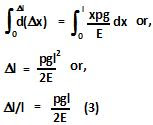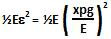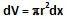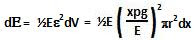## Friday, May 1, 2009

### Irodov Problem 1.310The part of the cylinder AX will be pulled downward at point X (which is at a height x from the bottom end B), by the weight of the cylinder in part XB given bywhere p is the density of the cylinder. Hence, the stress experienced at the cross-section of point X will beThus, at each height the cylinder experiences a different amount of stress. The strain at this point X is then given by,The net elongation of the cylinder can be found as,The elastic energy per unit volume at the location X is given by. Consider an infinitesimally thin disc at location X of thickness dx. The volume of this infinitesimally thin disc will be The elastic energy in an infinitesimally thin disc of thickness dx is given bygiven by,The total elastic energy contained in the cylinder is given by,